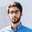Related Tags

python

# How to convert series to DataFrame in pandasNouman Abbasi

In pandas, converting a series to a DataFrame is a straightforward process.

pandas uses the to_frame() method to easily convert a series into a data frame.

## Syntax

Series.to_frame(name=None)


name:

• The passed name should substitute for the series name (if it has one).
• The fault is None
• Returns the DataFrame representation of Series

## Code

Let’s convert a pandas series s into a data frame and set the column name to 'Age':

import pandas as pd

s = pd.Series([25, 18, 40], name="vals")

df = s.to_frame(name="Age")

print(df)
print(type(df))

RELATED TAGS

python

CONTRIBUTORNouman Abbasi